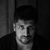# App Development

Announcements
Qlik Cloud Maintenance is scheduled between March 27-30. Visit Qlik Cloud Status page for more details.
cancel
Showing results for
Did you mean:Contributor II

## Nested If Then Else resulting in Performance Issue

Hi

I have a pivot table with 3 dimension and one measure.

Measure calculation consists of nested if else where it checking of each of the dimension and applying set analysis to get count of project code where flag > 0 and then also calculation percentage

With nested if else it takes more than 10 minutes , so i tried pick( match()) : it is taking even more time.

Can some one help me with solution?

If ( A='XYZ' or B='MNC'  ,

if( error_type='ABC_EDFG' , count(set_analysis to count the project_code where flag > 0 ) 'and Percentage'

coun( disticnt.... project code ) / total project code ,

if ,,,,,,,

so on for each of the error type.

3 RepliesCreator II

Hi ,

You could try moving the calculations to scripts and create flags , move the measure calculations in script .

ThanksSpecialist

hi,

if you dont mine can you share your input, table, expected output with  dummy example.

ksrinivasanContributor II
Author

I tried replacing the if then else with addition of each count function and applied the if condition inside of set analysis but some its not working.

count( distinct < set analysis ( error_type = 'A')  project_code ) + count(distinct <set analysis ( error_type = 'B') project code >)

but here it calculating the sum and then applying to all records irrespective of the error type .

e.g. error type A has count --> 5 and Error_type B has count 0

output

Error Type |    Count

A                            5

B                            5

C                            5

even thought B is 0 and no condition exists for type C the output is 5 for all records

Can someone please advice, the aim here is to replace if then else for better performance.

Original Calculation method

if( error_type = 'A', count(<set analysis  flag=0 > project_id) , if ( error_type = 'B', count(<set analysis  flag=0 >project_id) ,'')

Note : just the simplified version there are more conditions insideTags
Community Browser